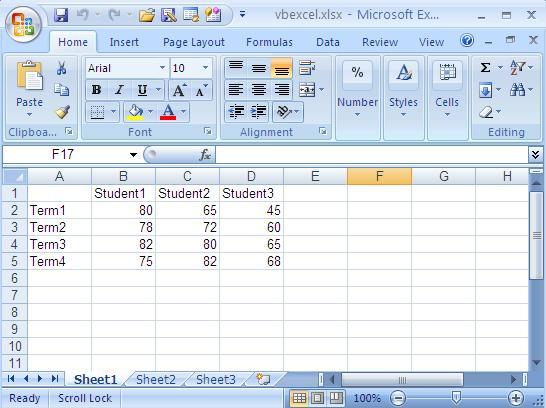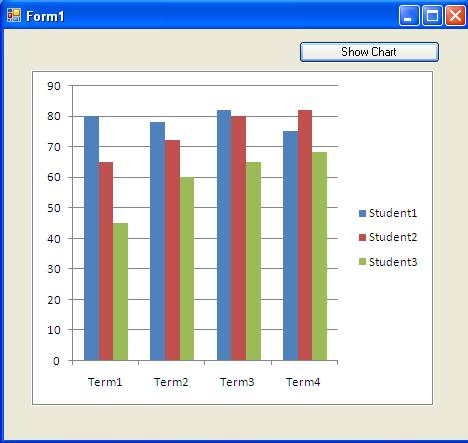VB.Net Tutorial

# How to Excel Chart in VB.NET Picture Box

The program presented below demonstrates how to obtain an Excel chart as an image and display it in a Picture Box. Prior to creating the chart, it is crucial to populate the Excel sheet with the required data. As you enter the data, the Excel sheet will gradually resemble the visual representation depicted in the following picture.

Once the data has been properly filled in, the next step involves creating a chart object in VB.NET. This chart object must be configured with essential information, including positions, size, data range, chart type, and other relevant attributes. By carefully adjusting these parameters, we can precisely tailor the chart to meet our specific needs and visual preferences.Following the chart configuration, the program proceeds to export the chart as a picture file. This entails utilizing the appropriate command to save the chart in a specific file format. Subsequently, the image file is loaded from its designated path into the Picture Box, allowing for direct visualization and interaction with the chart within the program's interface.

The resulting program screen, as illustrated in the accompanying picture, showcases the chart that has been successfully rendered and displayed within the Picture Box. This interactive and visually appealing representation enhances data comprehension and facilitates effective communication of insights and analysis.

The following picture shows the program screen after drawing the picture.Full Source VB.NET
Imports Excel = Microsoft.Office.Interop.Excel Public Class Form1 Private Sub Button1_Click(ByVal sender As System.Object, _ ByVal e As System.EventArgs) Handles Button1.Click Dim xlApp As Excel.Application Dim xlWorkBook As Excel.Workbook Dim xlWorkSheet As Excel.Worksheet Dim misValue As Object = System.Reflection.Missing.Value xlApp = New Excel.ApplicationClass xlWorkBook = xlApp.Workbooks.Add(misValue) xlWorkSheet = xlWorkBook.Sheets("sheet1") 'add data xlWorkSheet.Cells(1, 1) = "" xlWorkSheet.Cells(1, 2) = "Student1" xlWorkSheet.Cells(1, 3) = "Student2" xlWorkSheet.Cells(1, 4) = "Student3" xlWorkSheet.Cells(2, 1) = "Term1" xlWorkSheet.Cells(2, 2) = "80" xlWorkSheet.Cells(2, 3) = "65" xlWorkSheet.Cells(2, 4) = "45" xlWorkSheet.Cells(3, 1) = "Term2" xlWorkSheet.Cells(3, 2) = "78" xlWorkSheet.Cells(3, 3) = "72" xlWorkSheet.Cells(3, 4) = "60" xlWorkSheet.Cells(4, 1) = "Term3" xlWorkSheet.Cells(4, 2) = "82" xlWorkSheet.Cells(4, 3) = "80" xlWorkSheet.Cells(4, 4) = "65" xlWorkSheet.Cells(5, 1) = "Term4" xlWorkSheet.Cells(5, 2) = "75" xlWorkSheet.Cells(5, 3) = "82" xlWorkSheet.Cells(5, 4) = "68" 'create chart Dim chartPage As Excel.Chart Dim xlCharts As Excel.ChartObjects Dim myChart As Excel.ChartObject Dim chartRange As Excel.Range xlCharts = xlWorkSheet.ChartObjects myChart = xlCharts.Add(10, 80, 300, 250) chartPage = myChart.Chart chartRange = xlWorkSheet.Range("A1", "d5") chartPage.SetSourceData(Source:=chartRange) chartPage.ChartType = Excel.XlChartType.xlColumnClustered 'exporting chart as picture file xlWorkSheet.ChartObjects(1).chart.Export(FileName:= _ "C:\excel_chart_export.bmp", FilterName:="BMP") 'load the pipcture into the picture box PictureBox1.Image = New System.Drawing.Bitmap _ ("C:\excel_chart_export.bmp") xlWorkSheet.SaveAs("C:\vbexcel.xlsx") xlWorkBook.Close() xlApp.Quit() releaseObject(xlApp) releaseObject(xlWorkBook) releaseObject(xlWorkSheet) MsgBox("Chart File Exported !") End Sub Private Sub releaseObject(ByVal obj As Object) Try System.Runtime.InteropServices.Marshal.ReleaseComObject(obj) obj = Nothing Catch ex As Exception obj = Nothing Finally GC.Collect() End Try End SubEnd Class

By employing the techniques outlined in this program, we can seamlessly retrieve Excel charts as images and incorporate them into our VB.NET applications. This functionality enables us to work with the charts in a more versatile and interactive manner, supporting decision-making processes and facilitating data-driven discussions.

### Conclusion

Through the seamless integration of Excel charts as images into the program's interface, we enhance the overall user experience and promote a more engaging and intuitive environment for data exploration and analysis.